# Morley rank

(diff) ← Older revision | Latest revision (diff) | Newer revision → (diff)

An important notion and tool in model theory, a branch of mathematical logic. The Morley rank is an ordinal-valued dimension associated to first-order formulas with parameters from a modelof a complete first-order theory. It is defined inductively by: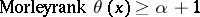if there is an elementary extensionofand infinitely many formulas(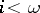) with parameters fromsuch that theare pairwise inconsistent,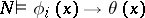for allandfor all. Fora limit ordinal (cf. also Ordinal number),if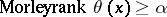for all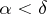. The Morley rank ofis said to be equal toif it is greater than or equal tobut not greater than or equal to. The Morley rank ofis said to be(or undefined) if it is not equal to any ordinal.

The Morley rank was introduced by M. Morley [a2] in his study of countable theoriessuch that for some uncountable cardinal number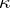,has a unique model of cardinality. Morley showed that a theorysatisfying the latter condition has a unique model of cardinalityfor any uncountable cardinal. An important part of his work was to show that every formula has ordinal-valued Morley rank. Subsequently, J.T. Baldwin [a1] showed that under Morley's hypothesis, every formula has finite Morley rank.

A classical example of Morley rank occurs in the (complete) theory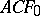of algebraically closed fields of characteristic. The fieldof complex numbers is a model, and for a formula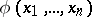with parameters indefining a non-singular algebraic variety, the Morley rank ofis precisely the dimension ofas a complex manifold.

Following the example of Morley rank, S. Shelah [a3] defined a host of rank-functions associated to formulas in first-order theories, which play an important role in classification theory.